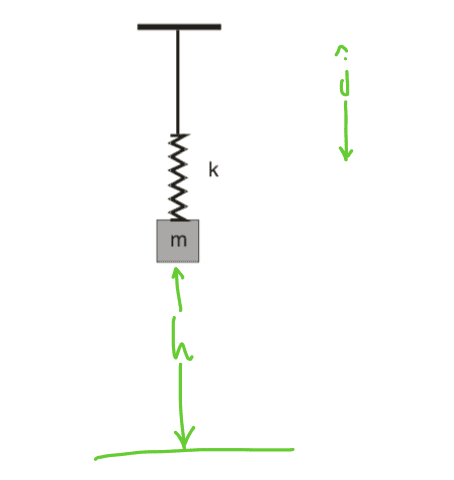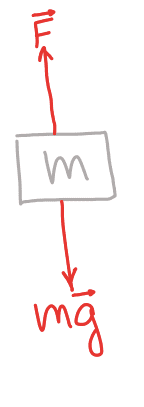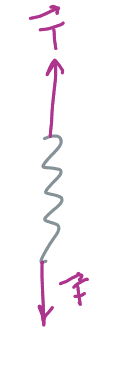# Find the tension of a rope with a mass and spring attached

valentina
Homework Statement:
An object of mass m is being held by a spring without mass and with constant k, which at the same time is attached to a inextensible rope without mass, attached to the ceiling.

At first the spring is at its natural length, and it's released with no velocity.

Find the maximum value for the tension of the rope.
Relevant Equations:
$$F=kx$$ (elastic force)

$$E_i=E_f$$
I'm having trouble with this problem, I think I solved it but I don't know if what I did is right...At first when the velocity is 0 and the spring is at its natural length, there's just gravitational potential energy, so $$E_i=mgh$$

And then, when the mass is released and then reaches its minimum height (I choose $$h=0$$ for this case), it only has elastic potential energy and for a moment it doesn't have kinetic energy (right?), so that must be $$E_f=\frac{kh^2}{2}$$

By the the theorem of conservation of mechanical energy, we have $$E_i=E_f$$, then $$mgh=\frac{kh^2}{2}$$, so $$kh=2mg$$

Then I draw the free body diagram for both the mass and for the spring, here's where I don't know if what I did was right:Where $$\vec{F}$$ is the elastic force

The equations I get are $$F=mg$$ and $$T=F$$ (because at that moment $$\vec{a}=0$$ ?)and this is where starts my confusion:

If I only use those two equations, I get that $$T=mg$$ BUT if I take into account the energy equation, I get that $$F=kh=2mg$$, and then $$T=2mg$$.

What would be the correct way to solve this problem? I'm really confused with the equations I got...

•Delta2

Homework Helper
Gold Member
because at that moment $$\vec{a}=0$$
Zero instantaneous velocity does not imply zero instantaneous acceleration. If a ball is thrown straight up and you consider the instant when the ball is at maximum height, what are the velocity and acceleration of the ball at that instant?

•Leo Liu
Homework Helper
Gold Member
What would be the correct way to solve this problem? I'm really confused with the equations I got...
The correct way to solve the problem is by ... (ahem) solving it correctly. Conservation of energy is one way. Drawing a free body diagram is another. If you draw the FBD, you need to understand that, for a spring-mass system, the acceleration is zero when the mass passes through the equilibrium position which is one amplitude away from the maximum extension (or compression) of the spring.

Homework Helper
Gold Member
...
Then I draw the free body diagram for both the mass and for the spring, here's where I don't know if what I did was right:

View attachment 275190View attachment 275191
Where $$\vec{F}$$ is the elastic force

The equations I get are ##F=mg## and ##T=F## (because at that moment $$\vec{a}=0$$ ?)and this is where starts my confusion:

If I only use those two equations, I get that $$T=mg$$ BUT if I take into account the energy equation, I get that $$F=kh=2mg$$, and then $$T=2mg$$.

What would be the correct way to solve this problem? I'm really confused with the equations I got...
I believe that your confusion started when assuming that ##F=mg## for height=0 in your diagram.
Rather, that force should be ##F=ma##.

The mass is experiencing two similar and mirroring conditions at both height=h and height=0: zero kinetic energy and maximum potential energy.

Maximum potential gravitational energy belongs to height=h.
Maximum potential elastic energy belongs to height=0.

There is a midpoint along that height at which all potential energy has become kinetic energy.
Because the mass has reached its highest velocity at that point, it can’t stop there.

The spring is still not acting on the mass at height=h; therefore, ##F=mg## is the only force inducing a downwards acceleration at that point.

The maximum value of the rope tension must be greater than mg for the mass to be stopped at height=0 and accelerated upwards again at same rate, in such way that a simple harmonic up-down motion is established.

http://hyperphysics.phy-astr.gsu.edu/hbase/shm.html#c1Last edited:
•Leo Liu and Delta2
Leo Liu
The equations I get are $$F=mg$$ and $$T=F$$ (because at that moment $$\vec{a}=0$$ ?)and this is where starts my confusion:
##F\neq mg## because you didn't take the acceleration into consideration. The correct answer, in my opinion, is ##F-mg=ma##. Also, the acceleration of the mass is not 0, which was pointed out by Tsny.

•etotheipi, Delta2 and Lnewqban